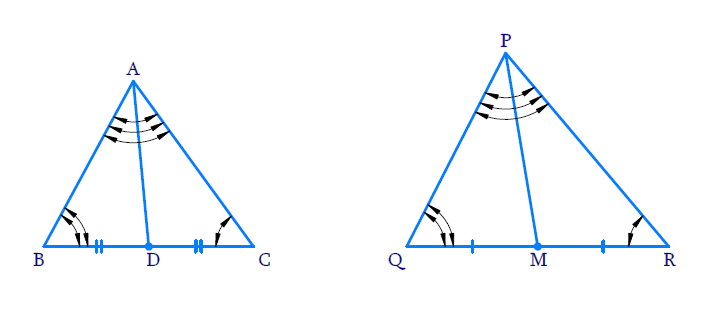# Ex.6.3 Q16 Triangles Solution - NCERT Maths Class 10

Go back to  'Ex.6.3'

## Question

If AD and PM are medians of triangles ABC and PQR, respectively where $$\Delta ABC\sim\Delta PQR$$, prove that$$\frac{AB}{PQ}=\frac{AD}{PM}$$·

Diagram## Text Solution

#### Reasoning:

As we know if one angle of a triangle is equal to one angle of the other triangle and the sides including these angles are proportional, then the two triangles are similar.

This is referred as $$SAS$$ (Side–Angle–Side) criterion for two triangles.

#### Steps:

$$\Delta A B C \sim \Delta P Q R$$

\begin{align}\Rightarrow \angle A B C=\angle P Q R \end{align}\qquad (corresponding angles) .........(1)

\begin{align}\Rightarrow \frac{A B}{P Q}=\frac{B C}{Q R}\end{align}\qquad  (corresponding sides)

\begin{align}& {\Rightarrow \frac{A B}{P Q}=\frac{B C / 2}{Q R / 2}}\end{align}

\begin{align}\Rightarrow \frac{AB}{PQ}=\frac{BD}{QM}\end{align}\qquad  (D and M are mid points of BC and QR) .......(2)

In $$\,\,\Delta ABD,\Delta PQM$$

$$\angle A B D=\angle P Q M$$ (from 1)

\begin{align}\frac{A B}{P Q}=\frac{B D}{Q M}\end{align}\qquad (from 2)

\begin{align}\Rightarrow \quad\Delta A B D \sim \Delta P Q M\end{align}\qquad  ($$SAS$$ criterion)

\begin{align}\Rightarrow\quad \frac{A B}{P Q}&=\frac{B D}{Q M}=\frac{A D}{P M}\end{align}\qquad (corresponding sides)

\begin{align} \Rightarrow \frac{A B}{P Q}&=\frac{A D}{P M}\end{align}

Learn from the best math teachers and top your exams

• Live one on one classroom and doubt clearing
• Practice worksheets in and after class for conceptual clarity
• Personalized curriculum to keep up with school